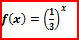Clutch Prep is now a part of Pearson
Ch.3: Pre-Calc (Part 3)WorksheetSee all chapters

# Exponential Functions

See all sections
Sections
Inverse Functions
Exponential Functions
Logarithmic Functions

Concept #1: Exponential Graphs, Rules of Exponents

Concept #2: Intro

Practice: Simplify the following.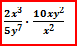Practice: Simplify the following.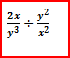Practice: Simplify the following.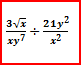Practice: Simplify the following.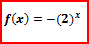Practice: Simplify the following.